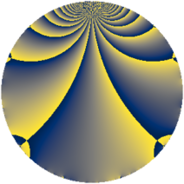# Properties

 Label 441.2.hLevel $441$ Weight $2$ Character orbit 441.h Rep. character $\chi_{441}(214,\cdot)$ Character field $\Q(\zeta_{3})$ Dimension $72$ Newform subspaces $8$ Sturm bound $112$ Trace bound $5$

# Learn more

## Defining parameters

 Level: $$N$$ $$=$$ $$441 = 3^{2} \cdot 7^{2}$$ Weight: $$k$$ $$=$$ $$2$$ Character orbit: $$[\chi]$$ $$=$$ 441.h (of order $$3$$ and degree $$2$$) Character conductor: $$\operatorname{cond}(\chi)$$ $$=$$ $$63$$ Character field: $$\Q(\zeta_{3})$$ Newform subspaces: $$8$$ Sturm bound: $$112$$ Trace bound: $$5$$ Distinguishing $$T_p$$: $$2$$, $$5$$

## Dimensions

The following table gives the dimensions of various subspaces of $$M_{2}(441, [\chi])$$.

Total New Old
Modular forms 128 88 40
Cusp forms 96 72 24
Eisenstein series 32 16 16

## Trace form

 $$72q + 2q^{2} + q^{3} + 66q^{4} - 5q^{5} + 2q^{6} - 12q^{8} - 7q^{9} + O(q^{10})$$ $$72q + 2q^{2} + q^{3} + 66q^{4} - 5q^{5} + 2q^{6} - 12q^{8} - 7q^{9} + 6q^{10} + 3q^{11} + 20q^{12} + 3q^{13} + 10q^{15} + 54q^{16} - 9q^{17} - 26q^{18} - 4q^{20} + 4q^{23} - 6q^{24} - 21q^{25} - 16q^{26} + 7q^{27} - 18q^{29} - 15q^{30} - 6q^{31} - 82q^{32} - 29q^{33} - 70q^{36} + 3q^{37} - 19q^{38} + 11q^{39} + 6q^{40} - 10q^{41} - 11q^{44} + 19q^{45} - 12q^{46} + 54q^{47} + 5q^{48} - 45q^{50} + 58q^{51} + 15q^{52} + 16q^{53} - q^{54} + 6q^{55} - 15q^{57} + 9q^{58} + 60q^{59} + 5q^{60} + 12q^{62} + 12q^{64} - 60q^{65} - 34q^{66} - 12q^{67} - 30q^{68} - 6q^{69} + 6q^{71} - 36q^{72} - 12q^{73} + 41q^{74} + 26q^{75} - 6q^{76} + 69q^{78} - 36q^{79} - 19q^{80} - 55q^{81} - 18q^{83} + 3q^{85} + 25q^{86} - 5q^{87} - 9q^{88} - 41q^{89} - 25q^{90} - 52q^{92} - 50q^{93} - 6q^{94} + 34q^{95} - 59q^{96} + 3q^{97} - 34q^{99} + O(q^{100})$$

## Decomposition of $$S_{2}^{\mathrm{new}}(441, [\chi])$$ into newform subspaces

Label Dim. $$A$$ Field CM Traces $q$-expansion
$$a_2$$ $$a_3$$ $$a_5$$ $$a_7$$
441.2.h.a $$2$$ $$3.521$$ $$\Q(\sqrt{-3})$$ None $$2$$ $$0$$ $$-1$$ $$0$$ $$q+q^{2}+(1-2\zeta_{6})q^{3}-q^{4}+(-1+\zeta_{6})q^{5}+\cdots$$
441.2.h.b $$6$$ $$3.521$$ 6.0.309123.1 None $$-2$$ $$-2$$ $$-5$$ $$0$$ $$q+(\beta _{2}+\beta _{3})q^{2}+(-\beta _{1}-\beta _{2}+\beta _{5})q^{3}+\cdots$$
441.2.h.c $$6$$ $$3.521$$ 6.0.309123.1 None $$-2$$ $$2$$ $$5$$ $$0$$ $$q+(\beta _{2}+\beta _{3})q^{2}+(\beta _{1}+\beta _{2}-\beta _{5})q^{3}+\cdots$$
441.2.h.d $$6$$ $$3.521$$ $$\Q(\zeta_{18})$$ None $$6$$ $$0$$ $$-3$$ $$0$$ $$q+(1-\zeta_{18}^{3}-\zeta_{18}^{4})q^{2}+(\zeta_{18}^{2}+\zeta_{18}^{5})q^{3}+\cdots$$
441.2.h.e $$6$$ $$3.521$$ $$\Q(\zeta_{18})$$ None $$6$$ $$0$$ $$3$$ $$0$$ $$q+(1-\zeta_{18}^{3}-\zeta_{18}^{4})q^{2}+(-\zeta_{18}^{2}+\cdots)q^{3}+\cdots$$
441.2.h.f $$10$$ $$3.521$$ 10.0.$$\cdots$$.1 None $$-4$$ $$1$$ $$-4$$ $$0$$ $$q+(-\beta _{1}+\beta _{5})q^{2}+\beta _{8}q^{3}+(1-\beta _{4}+\cdots)q^{4}+\cdots$$
441.2.h.g $$12$$ $$3.521$$ $$\mathbb{Q}[x]/(x^{12} - \cdots)$$ None $$4$$ $$0$$ $$0$$ $$0$$ $$q+\beta _{2}q^{2}+\beta _{10}q^{3}+(1+\beta _{2}+\beta _{4})q^{4}+\cdots$$
441.2.h.h $$24$$ $$3.521$$ None $$-8$$ $$0$$ $$0$$ $$0$$

## Decomposition of $$S_{2}^{\mathrm{old}}(441, [\chi])$$ into lower level spaces

$$S_{2}^{\mathrm{old}}(441, [\chi]) \cong$$ $$S_{2}^{\mathrm{new}}(63, [\chi])$$$$^{\oplus 2}$$